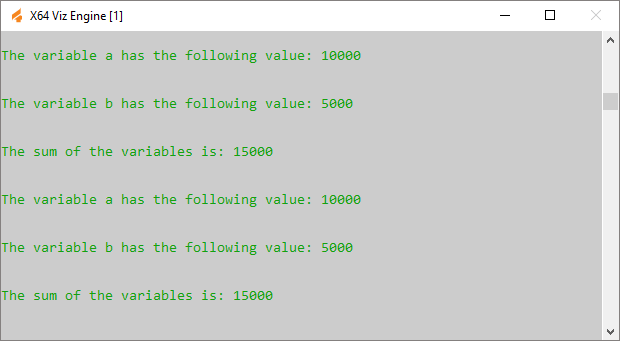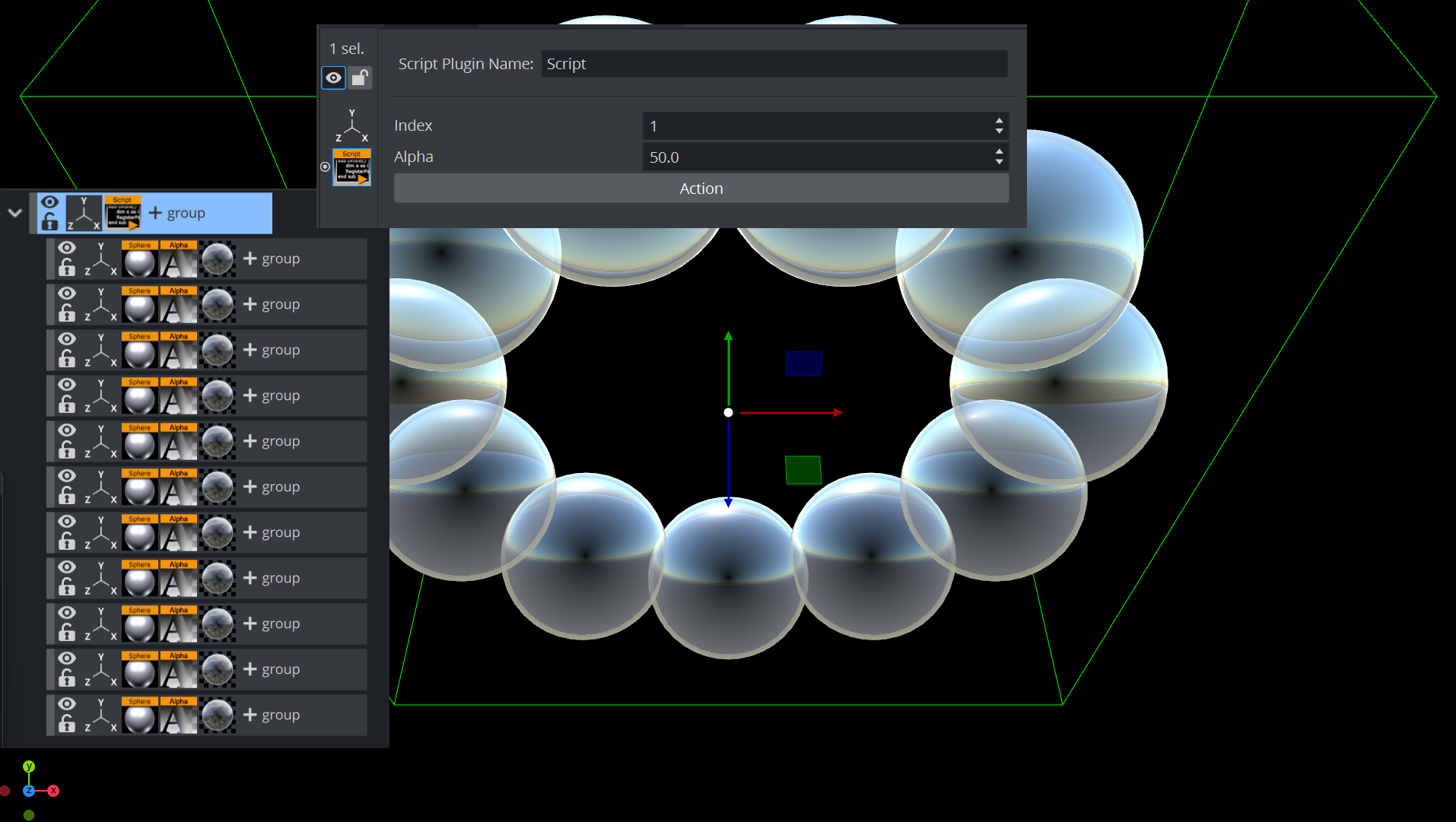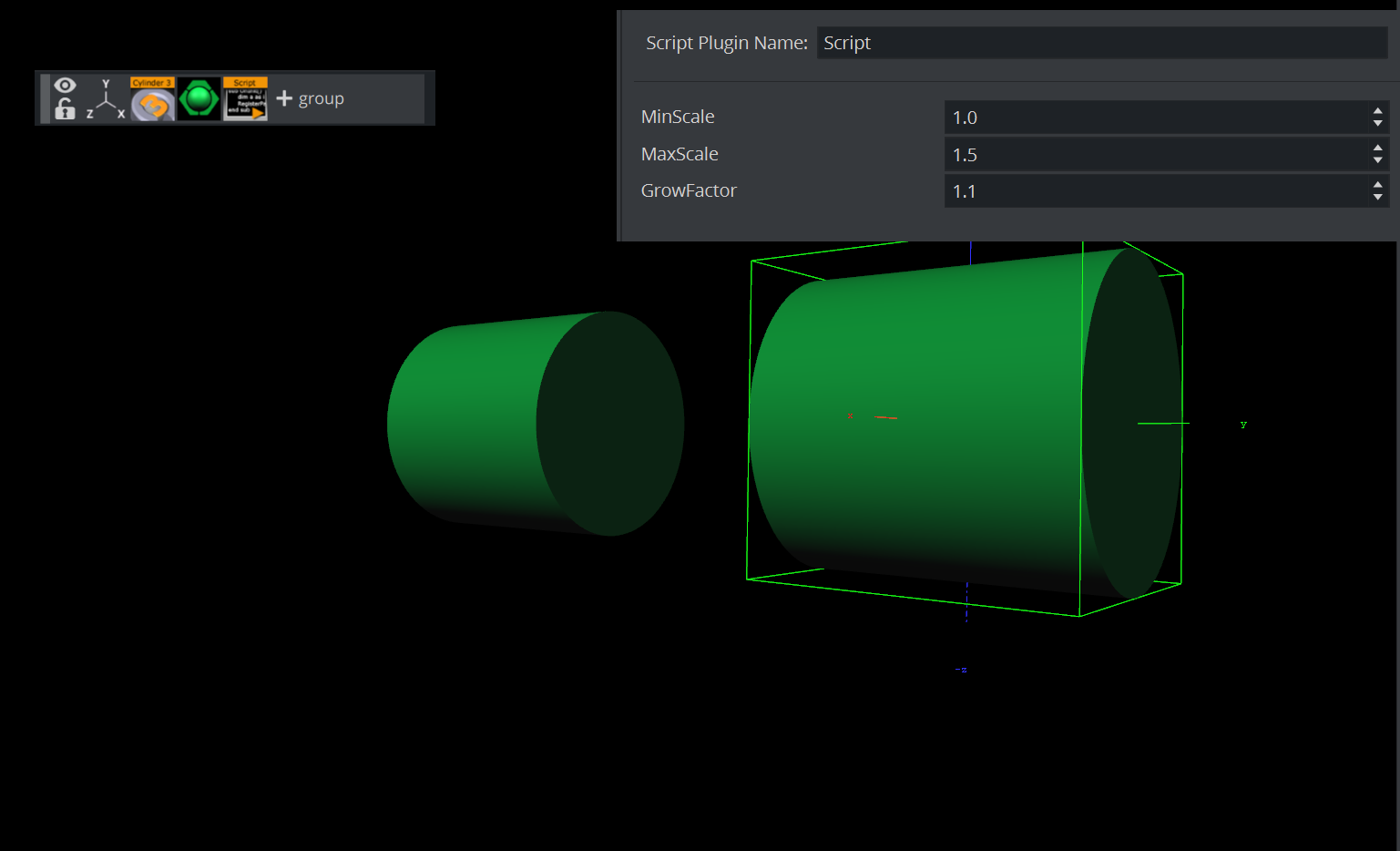## Viz Artist User Guide

Version 5.0 | Published December 20, 2022 ©

# Program Examples

To explain the described terms and definitions, this section shows some source code examples and explains the important steps and commands.

Most of the examples detailed here are written in an easy and understandable form for programing beginners. Some are basic, while others are more advanced and specific for their use case. More efficient code structures and improvements are possible.

This section contains information on the following topics:

# Initializing Scripts

This example declares and defines two variables with a value. In the main procedure OnInit() those two variables and their addition are output to the console using Println.

Callback procedures like OnInit() are used to initialize scripts.

`'Declaration of the variables `
`dim a as Integer `
`a = ``10000`` `
`dim b as Integer `
`b = ``5000`` `
`'Start of the main procedure sub OnInit() `
`'Executing the commands `
`println ``"\n"`` `
` println ``"The variable a has the following value: "`` & a `
`println ``"\n"`
` println ``"The variable b has the following value: "`` & b`
`println ``"\n"`
` println ``"The sum of the variables is: "`` & a + b `
`'End of the main procedure `
`end sub`

Result output to the Viz Engine console:# Register Parameters

This script example creates a custom editor for filling in index and alpha values. The index value decides which container does not receive the alpha value, whereas the alpha value is set for all other containers.`sub OnInitParameters()`
` RegisterParameterInt(``"index"``, ``"Index"``, ``1``, ``1``, ``7``)`
` RegisterParameterDouble(``"alpha"``, ``"Alpha"``, ``50.0``, ``0.0``, ``100.0``)`
` RegisterPushButton(``"action"``, ``"Action"``, ``1``) `
`end sub `
`sub OnExecAction(buttonId as Integer)`
` dim c as Container`
` dim i, index as Integer`
` dim a as Double`
` index = GetParameterInt(``"index"``)`
` a = GetParameterDouble(``"alpha"``)`
` i = ``1`
` c = ChildContainer`
` ``do`` ``while`` c <> ``null`
`     ``if`` i = index then`
`         c.alpha.value = ``100.0`
`     ``else`
`         c.alpha.value = a`
`     end ``if`
` i = i + ``1`
` c = c.NextContainer`
` loop `
`end sub`

# Mouse Events I (Set)

This script example shows how to use a ‘mouse event’” to set an object’s alpha value with OnEnter and OnLeave.

`sub OnEnter()`
` alpha.value = ``100.0`` `
`end sub `
`sub OnLeave()`
` alpha.value = ``50.0`` `
`end sub`

# Mouse Events II (Start, Stop and Reverse)

This script, when added to a container, allows an animation to be started with the click of a mouse button over an object (for example, rectangle). Press again and it stops. Press again and it continues from the point it stopped.

`dim my_dir = stage.FindDirector(``"Default"``) `
`sub OnLButtonDown()`
` ``if`` my_dir.IsAnimationRunning() then`
` my_dir.StopAnimation()`
` ``else`
` my_dir.ContinueAnimation()`
` end ``if`` `
`end sub`

With the script detailed below, start an animation and let it run until a stop point. Click again and it runs in reverse:

`dim my_dir = stage.FindDirector(``"Defult"``) `
`sub OnLButtonDown()`
` menu.ContinueAnimation()`
` ``if`` my_dir.reverse == ``true`` then`
` my_dir.reverse = ``false`
` ``else`
` my_dir.reverse = ``true`
` end ``if`` `
`end sub`

# Mouse Events III (Grow and Shrink)

With this script added to a Container an object grows when the mouse is hovering over the object and conversely. In this case, the center of the object is set to the lower right corner to let it grow out from that center.`dim growing as Boolean = ``false`` `
`sub OnInitParameters()`
` RegisterParameterDouble(``"min"``, ``"MinScale"``, ``1.0``, ``0.0``, ``10.0``)`
` RegisterParameterDouble(``"max"``, ``"MaxScale"``, ``1.5``, ``0.0``, ``10.0``)`
` RegisterParameterDouble(``"fac"``, ``"GrowFactor"``, ``1.1``, ``1.0``, ``10.0``) `
`end sub `
`sub OnEnter()`
` growing = ``true`` `
`end sub `
`sub OnLeave()`
` growing = ``false`` `
`end sub `
`sub OnExecPerField()`
` dim min = GetParameterDouble(``"min"``)`
` dim max = GetParameterDouble(``"max"``)`
` dim fac = GetParameterDouble(``"fac"``)`
` ``if`` growing then`
` scaling.xyz *= fac`
` ``if`` scaling.x > max then scaling.xyz = max`
` ``else`
` scaling.xyz /= fac`
` ``if`` scaling.x < min then scaling.xyz = min`
` end ``if`` `
`end sub`

# Simple Gesture Recognition

This example script can be used to detect a drawn square on the screen:

`dim gRecognizer as gesturerecognizer `
`sub OnInit()`
` gRecognizer.handlepatterns = ``true`
` gRecognizer.addhandler(scriptplugininstance) `
`end sub `
` `
`sub OnGesture(gesture As Gesture)`
` ``if`` gesture.patternid == PATTERN_SQUARE then`
` println(``"This is a square"``)`
` end ``if`` `
`end sub`
` `
`sub OnTouchTrace(trace as Trace, touch as Touch)`
` gRecognizer.addtrace(trace) `
`end sub`

Note: Some gesture patterns need to be checked for their alignment as well, for example up, down, left, and so on. For this you can activate the CheckPatternOrientation property in the gesture recognizer object.

# Calculate the Days of a Week and Month

This script example calculates the day of the week and the number of days of the month:

`DIM smap AS Array[String]`
` `
`smap.size = ``7`
`smap[``0``] = ``"Sun"`
`smap[``1``] = ``"Mon"`
`smap[``2``] = ``"Tue"`
`smap[``3``] = ``"Wed"`
`smap[``4``] = ``"Thu"`
`smap[``5``] = ``"Fri"`
`smap[``6``] = ``"Sat"`
` `
`function CalcDayOfWeek(iYear AS Integer, iMonth AS Integer, iDay AS Integer) AS String`
` `
`DIM dt AS DateTime`
` dt.year = iYear`
` dt.month = iMonth`
` dt.dayofmonth = iDay`
` dt.normalize()`
` `
` CalcDayOfWeek = smap[dt.dayofweek] `
`end function `
` `
`function CalcNumOfDaysOfMonth(iYear AS Integer, iMonth AS Integer) AS Integer`
`DIM dt AS DateTime`
` dt.year = iYear`
` dt.month = iMonth+``1`
` dt.dayofmonth = ``0`
` dt.normalize()`
` CalcNumOfDaysOfMonth = dt.dayofmonth`
`end function `
` `
`sub OnInit()`
`DIM dt AS DateTime`
` dt.year = ``2019`
` dt.month = ``2`
` dt.dayofmonth = ``23`
` `
` println(``"Date: "`` & CalcDayOfWeek(dt.year, dt.month, dt.dayofmonth) & ``", "`` & dt.year & ``""`` & dt.month & ``""`` & dt.dayofmonth)`
` println(``"Days: "`` & CalcNumOfDaysOfMonth(dt.year, dt.month)) `
`end sub`

# Update a Scene Using a Text File

This script example updates a Scene from a text file.

`dim timer,limit as integer `
`timer = ``0`` `
`sub OnInitParameters()`
` RegisterInfoText(``"Text File Grabber 1.0"`` & chr(``10``) & chr(``10``) `
` & ``"Grabs text file and puts optional prefix/suffix around the contents of the text file."`` & chr(``10``) `
` & ``"Filename supports paths with drive letters and UNC paths."`` & chr(``10``) & chr(``10``) `
` & ``"Text in \"Default Text\" box is used if file specified in File box cannot be opend or accessed."`` & chr(``10``) & chr(``10``) `
` & ``"Listens to scene map variable \"text_update\" -- text files are re-read when this variable changes."``)`
` RegisterFileSelector(``"file"``, ``"File"``, ``"c:\test"``, ``"1.txt"``, ``"*.*"``)`
` RegisterParameterString(``"default"``,``"Default text"``,``""``,``40``,``50``,``""``)`
` RegisterParameterString(``"prefix"``,``"Prefix (optional)"``,``""``,``40``,``50``,``""``) `
` RegisterParameterBool(``"prefixIfNull"``,``"Include prefix if file not found"``,FALSE)`
` RegisterParameterString(``"suffix"``,``"Suffix (optional)"``,``""``,``40``,``50``,``""``)`
` RegisterParameterBool(``"suffixIfNull"``,``"Include suffix if file not found"``,FALSE)`
` RegisterParameterInt(``"updateFields"``,``"Auto-Update interval (fields)"``,``300``,``60``,``999999999999``)`
` RegisterParameterBool(``"debug"``,``"Debug output to console"``,FALSE) `
`end sub `
` `
`sub OnParameterChanged(parameterName As String) `
`  're-populate text when a parameter changes`
` ``if`` parameterName <> ``"updateFields"`` then UpdateText `
` ``if`` parameterName = ``"updateFields"`` then`
` limit = GetParameterInt(``"updateFields"``)`
` end ``if`` `
`end sub `
` `
`sub OnExecPerField()`
` timer = timer + ``1`
` ``if`` timer >= limit then`
` ``if`` GetParameterBool(``"debug"``) then println ``"Timer limit reached"`
` UpdateText`
` timer = ``0`
` end ``if`` `
`end sub `
` `
`sub OnInit()`
` Scene.Map.RegisterChangedCallback(``"text_update"``)`
` limit = GetParameterInt(``"updateFields"``)`
` UpdateText `
`end sub `
` `
`sub OnSharedMemoryVariableChanged(map As SharedMemory, mapKey As String)`
` ``if`` mapKey = ``"text_update"`` then`
` UpdateText`
` end ``if`` `
`end sub `
` `
`sub UpdateText()`
` geometry.text = GetTextFile() `
`end sub`
` `
`function GetTextFile() as string`
` dim content, path as string`
` path = GetParameterString(``"file"``)`
` ``if`` GetParameterBool(``"debug"``) then println ``"File path: "`` & path`
` ``if`` System.LoadTextFile( path, content ) then`
` ``if`` GetParameterBool(``"debug"``) then println ``"Successfully loaded file"`
` content.trim`
` ``if`` len(GetParameterString(``"prefix"``)) > ``0`` then content = GetParameterString(``"prefix"``) & ``" "`` & content`
` ``if`` len(GetParameterString(``"suffix"``)) > ``0`` then content = content & ``" "`` & GetParameterString(``"suffix"``)`
` ``else`
` ``if`` GetParameterBool(``"debug"``) then println ``"Could not load file; using default text"`
` content = GetParameterString(``"default"``)`
` ``if`` GetParameterBool(``"prefixIfNull"``) then content = GetParameterString(``"prefix"``) & ``" "`` & content`
` ``if`` GetParameterBool(``"suffixIfNull"``) then content = content & ``" "`` & GetParameterString(``"suffix"``)`
` end ``if`
` GetTextFile = content`
` ``if`` GetParameterBool(``"debug"``) then println ``"Returning '"`` & GetTextFile & ``"'"`` `
`end function`